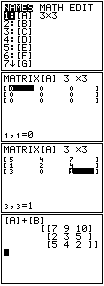Go to contentHow to add two 3 x 3 matrices on a GDC (graphical display calculator). This explanation uses the TI-82/83/84 range of calculators.

The calculator has memory slots set aside for matrices: [A], [B], ..., [J].
1. Press the MATRIX key. This brings you to a menu with a list of all the matrices.
2. Use the right-arrow twice to move over to EDIT so that you can create a matrix.
3. Type a 1 (or ENTER) for matrix [A].
4. Press 3 ENTER 3 ENTER to indicate a 3x3 matrix.
5. Now choose values of the elements of the matrix until you've filled the matrix.
7. Repeat the last 4 steps to create matrix B.
8. Press MATRIX then ENTER
9. Press the + button
10. Press MATRIX then 2 then ENTER

A display of the sum A + B will be shown. Use the cursor keys to move about the matrix to see all the entries.You can get a better display of the maths by downloading special TeX fonts from jsMath. In the meantime, we will do the best we can with the fonts you have, but it may not be pretty and some equations may not be rendered correctly.

## Glossary

### gdc

graphic display calculator

### matrix

a rectangular or square grid of numbers.

### mode

the value in a set of discrete data that occurs most frequently

### range

In Statistics: the difference between the largest and smallest values in a data set; a simple measure of spread or variation
In Pure Maths: the values that y can take given an equation y=f(x) and a domain for x.

### union

The union of two sets A and B is the set containing all the elements of A and B.

Full Glossary List

## This question appears in the following syllabi:

SyllabusModuleSectionTopicExam Year
AQA A-Level (UK - Pre-2017)FP4Matrix algebra3x3 matrices-
AQA A2 Further Maths 2017Pure MathsFurther Matrices3x3 Matrices-
AQA AS/A2 Further Maths 2017Pure MathsFurther Matrices3x3 Matrices-
CBSE XII (India)AlgebraDeterminantsDeterminant of a square matrix (up to 3 x 3)-
CCEA A-Level (NI)FP1Matrix algebra3x3 matrices-
Edexcel A-Level (UK - Pre-2017)FP3Matrix algebra3x3 matrices-
Edexcel AS Further Maths 2017Core Pure MathsMatrices3x3 Matrices-
Edexcel AS/A2 Further Maths 2017Core Pure MathsMatrices3x3 Matrices-
Methods (UK)M5Matrix algebra3x3 matrices-
OCR A-Level (UK - Pre-2017)FP1Matrix algebra3x3 matrices-
OCR AS Further Maths 2017Pure CoreDeterminants, Inverses and Equations3x3 Matrices-
OCR MEI A2 Further Maths 2017Core Pure BMatrices and Transformations3x3 Matrices-
OCR-MEI A-Level (UK - Pre-2017)FP1Matrix algebra3x3 matrices-
Pre-Calculus (US)E2Matrix algebra3x3 matrices-